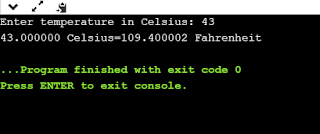## C program to Convert Celsius to Fahrenheit

Hey guys welcome back, hope you are enjoying other blogs,so in this Tutorial i am explaining how
to convert Celsius to Fahrenheit using language c.

For example if you give Fahrenheit as 43,then it will print it in Celsius.

This C++ Program performs Celsius to Fahrenheit conversion. The program reads the temperature in Celsius, multiplies the temperature in Celsius by 9 / 5 and adds 32 to obtain temperature in Fahrenheit. Here is source code of the C++ program which performs Celsius to Fahrenheit conversion.

CODE

#include <stdio.h> int main() { float celsius, fahrenheit; printf("Enter temperature in Celsius: "); scanf("%f", &celsius); fahrenheit = (celsius * 9 / 5) + 32;
printf("%f Celsius=%f Fahrenheit",celsius,fahrenheit);
return 0; }

OUTPUT